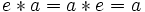# Binary operation on magma determines neutral element

(diff) ← Older revision | Latest revision (diff) | Newer revision → (diff)
Suppose$(S,*)$ is a magma (set$S$ with associative binary operation$*$). Then, if there exists a neutral element for$*$ (i.e., an element$e$ such that$e * a = a * e = a$ for all$a \in S$), the element$e$ is uniquely determined by$*$.